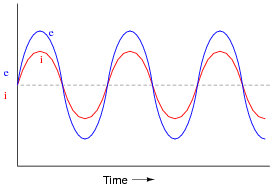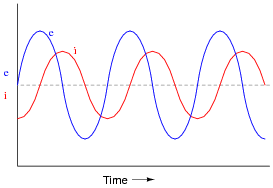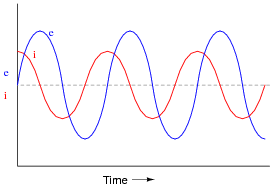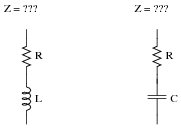# Impedance

## AC Electric Circuits

• #### Question 1

 Don’t just sit there! Build something!!

Learning to mathematically analyze circuits requires much study and practice. Typically, students practice by working through lots of sample problems and checking their answers against those provided by the textbook or the instructor. While this is good, there is a much better way.

You will learn much more by actually building and analyzing real circuits, letting your test equipment provide the “answers” instead of a book or another person. For successful circuit-building exercises, follow these steps:

1. Carefully measure and record all component values prior to circuit construction.
2. Draw the schematic diagram for the circuit to be analyzed.
3. Carefully build this circuit on a breadboard or other convenient medium.
4. Check the accuracy of the circuit’s construction, following each wire to each connection point, and verifying these elements one-by-one on the diagram.
5. Mathematically analyze the circuit, solving for all voltage and current values.
6. Carefully measure all voltages and currents, to verify the accuracy of your analysis.
7. If there are any substantial errors (greater than a few percent), carefully check your circuit’s construction against the diagram, then carefully re-calculate the values and re-measure.

For AC circuits where inductive and capacitive reactances (impedances) are a significant element in the calculations, I recommend high quality (high-Q) inductors and capacitors and powering your circuit with low-frequency voltage (power-line frequency works well) to minimize parasitic effects. If you are on a restricted budget, I have found that inexpensive electronic musical keyboards serve well as “function generators” for producing a wide range of audio-frequency AC signals. Be sure to choose a keyboard “voice” that closely mimics a sine wave (the “panflute” voice is typically good), if sinusoidal waveforms are an important assumption in your calculations.

As usual, avoid very high and very low resistor values, to avoid measurement errors caused by meter “loading”. I recommend resistor values between 1 kΩ and 100 kΩ.

One way you can save time and reduce the possibility of error is to begin with a very simple circuit and incrementally add components to increase its complexity after each analysis, rather than building a whole new circuit for each practice problem. Another time-saving technique is to re-use the same components in a variety of different circuit configurations. This way, you won’t have to measure any component’s value more than once.

• #### Question 2

In DC circuits, we have Ohm’s Law to relate voltage, current, and resistance together:

 E = I R

In AC circuits, we similarly need a formula to relate voltage, current, and impedance together. Write three equations, one solving for each of these three variables: a set of Ohm’s Law formulae for AC circuits. Be prepared to show how you may use algebra to manipulate one of these equations into the other two forms.

• #### Question 3

In this AC circuit, the resistor offers 300 Ω of resistance, and the inductor offers 400 Ω of reactance. Together, their series opposition to alternating current results in a current of 10 mA from the 5 volt source:How many ohms of opposition does the series combination of resistor and inductor offer? What name do we give to this quantity, and how do we symbolize it, being that it is composed of both resistance (R) and reactance (X)?

• #### Question 4

In this AC circuit, the resistor offers 3 kΩ of resistance, and the capacitor offers 4 kΩ of reactance. Together, their series opposition to alternating current results in a current of 1 mA from the 5 volt source:How many ohms of opposition does the series combination of resistor and capacitor offer? What name do we give to this quantity, and how do we symbolize it, being that it is composed of both resistance (R) and reactance (X)?

• #### Question 5

While studying DC circuit theory, you learned that resistance was an expression of a component’s opposition to electric current. Then, when studying AC circuit theory, you learned that reactance was another type of opposition to current. Now, a third term is introduced: impedance. Like resistance and reactance, impedance is also a form of opposition to electric current.

Explain the difference between these three quantities (resistance, reactance, and impedance) using your own words.

• #### Question 6

It is often necessary to represent AC circuit quantities as complex numbers rather than as scalar numbers, because both magnitude and phase angle are necessary to consider in certain calculations.

When representing AC voltages and currents in polar form, the angle given refers to the phase shift between the given voltage or current, and a “reference” voltage or current at the same frequency somewhere else in the circuit. So, a voltage of 3.5 V ∠−45o means a voltage of 3.5 volts magnitude, phase-shifted 45 degrees behind (lagging) the reference voltage (or current), which is defined to be at an angle of 0 degrees.

But what about impedance (Z)? Does impedance have a phase angle, too, or is it a simple scalar number like resistance or reactance?

Calculate the amount of current that would go through a 100 mH inductor with 36 volts RMS applied to it at a frequency of 400 Hz. Then, based on Ohm’s Law for AC circuits and what you know of the phase relationship between voltage and current for an inductor, calculate the impedance of this inductor in polar form. Does a definite angle emerge from this calculation for the inductor’s impedance? Explain why or why not.

• #### Question 7

Express the impedance (Z) in both polar and rectangular forms for each of the following components:

A resistor with 500 Ω of resistance
An inductor with 1.2 kΩ of reactance
A capacitor with 950 Ω of reactance
A resistor with 22 kΩ of resistance
A capacitor with 50 kΩ of reactance
An inductor with 133 Ω of reactance
• #### Question 8

Real inductors and capacitors are never purely reactive. There will inevitably be some resistance intrinsic to these devices as well.

Suppose an inductor has 57 Ω of winding resistance, and 1500 Ω of reactance at a particular frequency. How would this combination be expressed as a single impedance? State your answer in both polar and rectangular forms.

• #### Question 9

Not only do reactive components unavoidably possess some parasitic (“stray”) resistance, but they also exhibit parasitic reactance of the opposite kind. For instance, inductors are bound to have a small amount of capacitance built-in, and capacitors are bound to have a small amount of inductance built-in. These effects are not intentional, but they exist anyway.

Describe how a small amount of capacitance comes to exist within an inductor, and how a small amount of inductance comes to exist within a capacitor. Explain what it is about the construction of these two reactive components that allows the existence of “opposite” characteristics.

• #### Question 10

Suppose you were given a component and told it was either a resistor, an inductor, or a capacitor. The component is unmarked, and impossible to visually identify. Explain what steps you would take to electrically identify what type of component it was, and what its value was, without the use of any test equipment except a signal generator, a multimeter (capable of measuring nothing but voltage, current, and resistance), and some miscellaneous passive components (resistors, capacitors, inductors, switches, etc.). Demonstrate your technique if possible.

• #### Question 11

Suppose you were given two components and told one was an inductor while the other was a capacitor. Both components are unmarked, and impossible to visually distinguish or identify. Explain how you could use an ohmmeter to distinguish one from the other, based on each component’s response to direct current (DC).

Then, explain how you could approximately measure the value of each component using nothing more than a sine-wave signal generator and an AC meter capable only of precise AC voltage and current measurements across a wide frequency range (no direct capacitance or inductance measurement capability), and show how the reactance equation for each component (L and C) would be used in your calculations.

• #### Question 12

If a sinusoidal voltage is applied to an impedance with a phase angle of 0o, the resulting voltage and current waveforms will look like this:Given that power is the product of voltage and current (p = i e), plot the waveform for power in this circuit.

• #### Question 13

If a sinusoidal voltage is applied to an impedance with a phase angle of 90o, the resulting voltage and current waveforms will look like this:Given that power is the product of voltage and current (p = i e), plot the waveform for power in this circuit. Also, explain how the mnemonic phrase “ELI the ICE man” applies to these waveforms.

• #### Question 14

If a sinusoidal voltage is applied to an impedance with a phase angle of -90o, the resulting voltage and current waveforms will look like this:Given that power is the product of voltage and current (p = i e), plot the waveform for power in this circuit. Also, explain how the mnemonic phrase “ELI the ICE man” applies to these waveforms.

• #### Question 15

Speakers used for audio reproduction systems (stereos, public address systems, etc.) act as power loads to the amplifiers which drive them. These devices convert electrical energy into sound energy, which then dissipates into the surrounding air. In this manner, a speaker acts much like a resistor: converting one form of energy (electrical) into another, and then dissipating that energy into the surrounding environment. Naturally, it makes sense to describe the nature of such loads in units of “ohms” (Ω), so that they may be mathematically analyzed in a manner similar to resistors.

Yet, despite the dissipative nature of audio speakers, their “ohms” rating is specified as an impedance rather than a resistance or a reactance. Explain why this is.

• #### Question 16

Engineers often write the capacitive and inductive reactance formulae in a different way from what you may have seen:

 XL = ωL

 XC = 1 ωC

These equations should look familiar to you, from having seen similar equations containing a term for frequency (f). Given these equations’ forms, what is the mathematical definition of ω? In other words, what combination of variables and constants comprise “ω”, and what unit is it properly expressed in?

• #### Question 17

Engineers often calculate the impedance of pure capacitances and pure inductances in a way that directly provides results in rectangular (complex) form:

 ZL = j ωL

 ZC = −j 1 ωC

The bold-faced type (Z instead of Z) signifies the calculated impedance as a complex rather than a scalar quantity. Given these equations’ forms, what is the mathematical definition of ω? In other words, what combination of variables and constants comprise “ω”, and what unit is it properly expressed in?

Also, determine what the equations would look like for calculating the impedance of these series networks:• #### Question 18

The mathematical inverse, or reciprocal, of resistance (R) is a quantity called conductance (G).

 G = 1 R

Is there an equivalent quantity for impedance (Z)? What is the reciprocal of impedance, and what unit of measurement is it expressed in? Hint: its symbol is Y.

Is there an equivalent quantity for reactance (X)? What is the reciprocal of reactance, and what unit of measurement is it expressed in? Hint: its symbol is B.

• #### Question 19

The mathematical inverse, or reciprocal, of resistance (R) is a quantity called conductance (G).

 G = 1 R

Is there an equivalent quantity for reactance? What is the reciprocal of reactance, and what unit of measurement is it expressed in? Hint: its symbol is B.